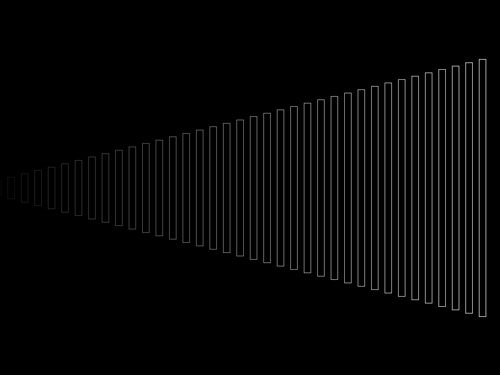CategoriesAnother Processing sketch, fairly simple. This one does introduce a function (or subroutine) named ‘makeBox’ that we pass some values into.

You can also see where we used height/2 which is the proper way to center something on the screen, rather than taking the 600 we specified and just using 300 as the halfway point.

```/*
* 20110509.pde
*/

void setup() {
size(800, 600);
background(0);
stroke(255);
noFill();
}

void draw() {

for (int xVal = 5; xVal < (width - 20); xVal = xVal + 20) {
int myWidth = 10;
int myHeight = xVal/2;
int yVal = (height/2) - (myHeight/2);
int myColor = xVal/4;

makeBox(xVal, yVal, myWidth, myHeight, myColor);

}

}

void makeBox(int x, int y, int w, int h, int c) {
stroke(c);
rect(x, y, w, h);
}
```

(These sketches may not be all that impressive, but it’s giving me a chance to flex my programming muscles in Processing.)